• MATLAB在已有shape文件基础上画地图，出图；想求一份亚洲后者师姐的shape文件，特出程序
• 利用MATLAB自带的地图工具箱画地图，并举例子说明
• matlab画地图，可画四川地图，世界地图，亚洲地图
• ## 利用matlab画地图

万次阅读 多人点赞 2018-09-30 13:08:16
目录 方法一：利用 GIS画图 方法二：利用地图工具箱画图 方法一：利用 GIS画图 中国国家基础地理信息系统（GIS）... matlab地图工具箱其他作图效果如下 欢迎加群获取数据 https://www.jianshu.com/p/cb3ccbae9c88
目录

方法一：利用 GIS画图

方法二：利用地图工具箱画图

方法一：利用 GIS画图

中国国家基础地理信息系统（GIS）数据

Geographic Information System (GIS) Basic Data of China中国国家基础地理信息系统（GIS）数据，包括中国省级、县级行政界线、各级河流水系、主要公路铁路、世界行政边界图等矢量数据，可打包下载。该数据集是在国家基础地理信息系统网站改版之前下载获取。数据下载地址。或者

地球物理相关资源

各国地区shp文件下载

世界行政区划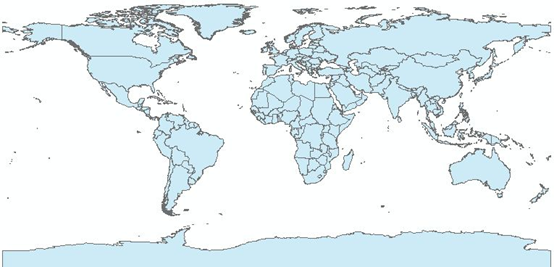中国省级行政区划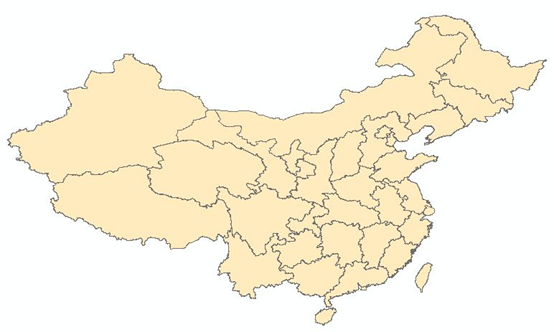中国县级行政界线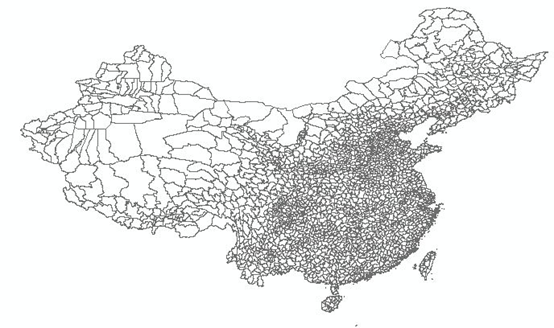中国县级居民点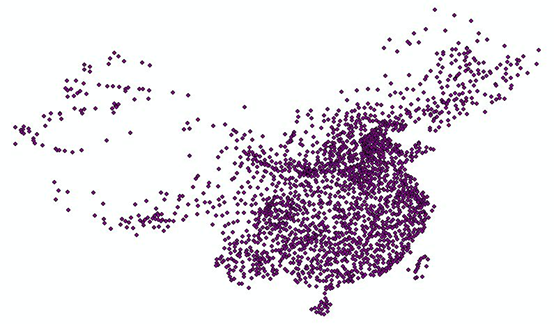南海诸岛及九段线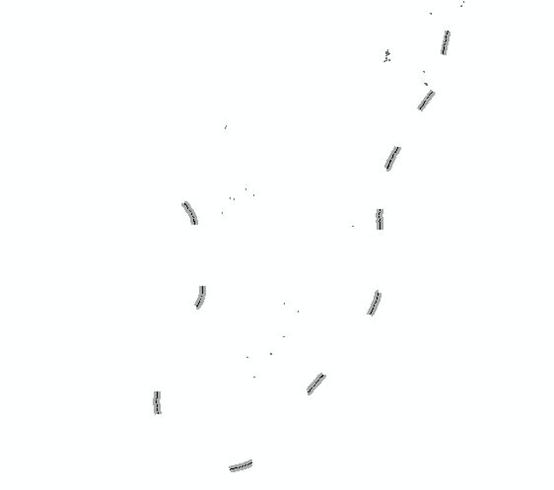一级河流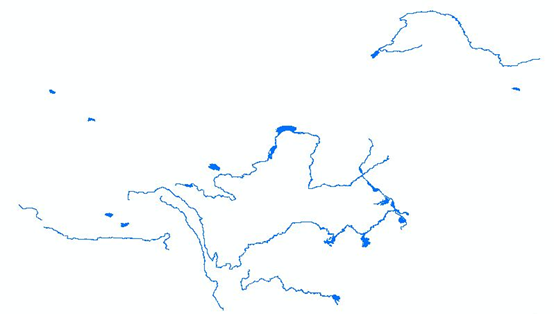三级河流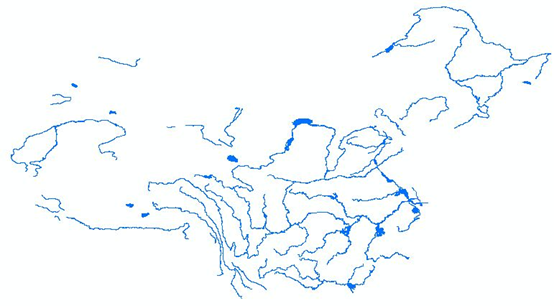五级河流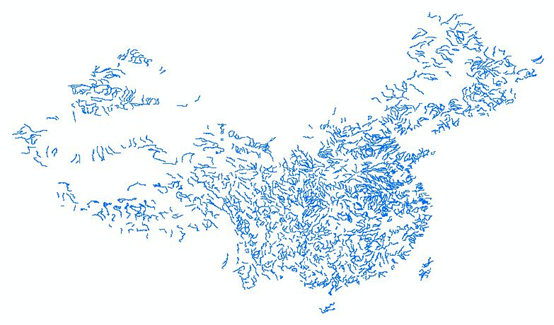主要铁路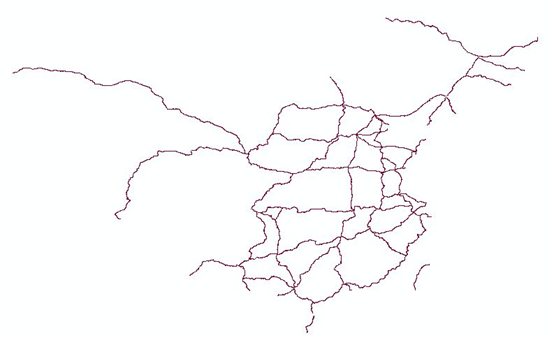主要公路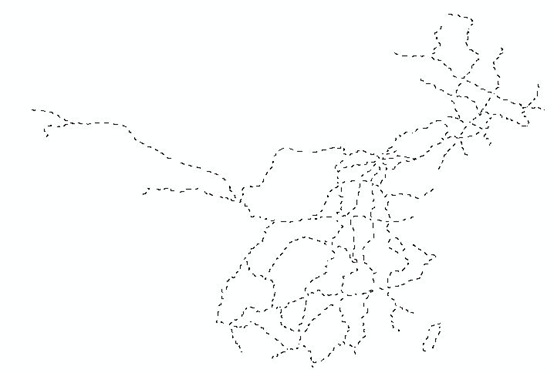方法二：利用地图工具箱画图

close all; clc
m_proj('lambert','long',[135,73],'lat',[10,53]);%经纬度
m_coast('color','b','linewidth',2);%画海岸线
m_coast('patch',[1.0,0.85,0.7]);
m_elev('contourf',[500:500:6000]);
colormap('jet(30)')
colorbar('location','southoutside');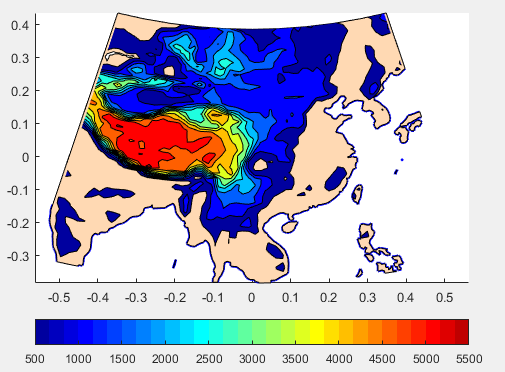matlab地图工具箱其他作图效果如下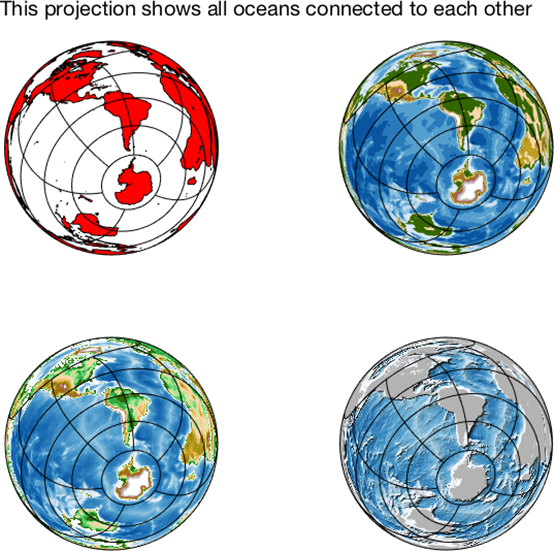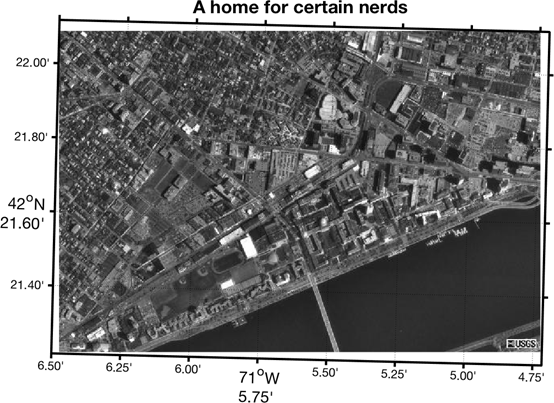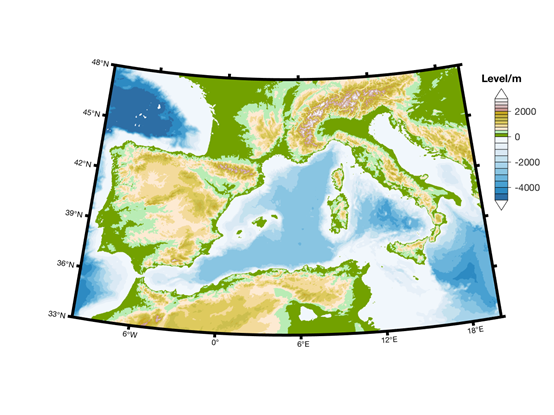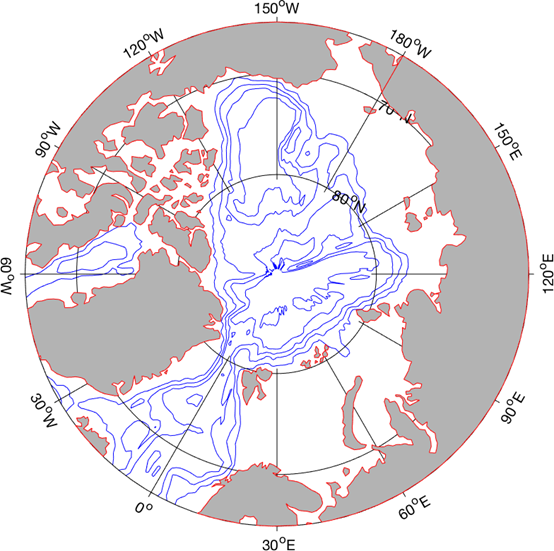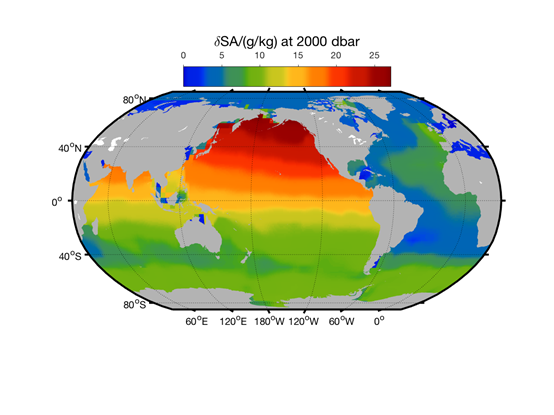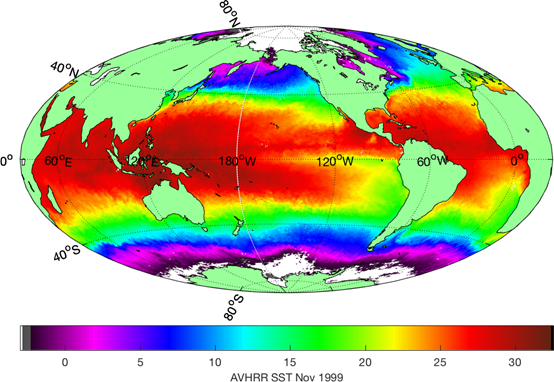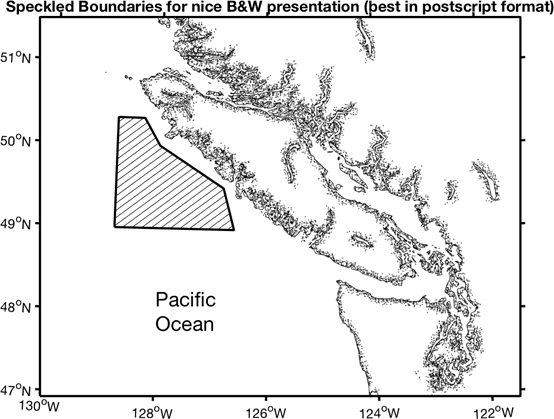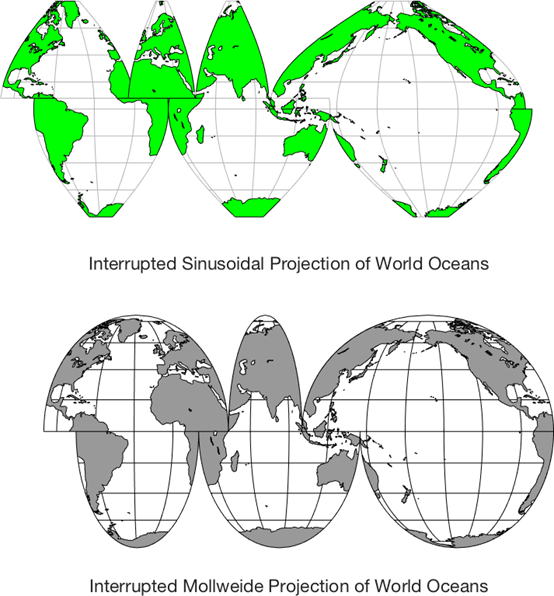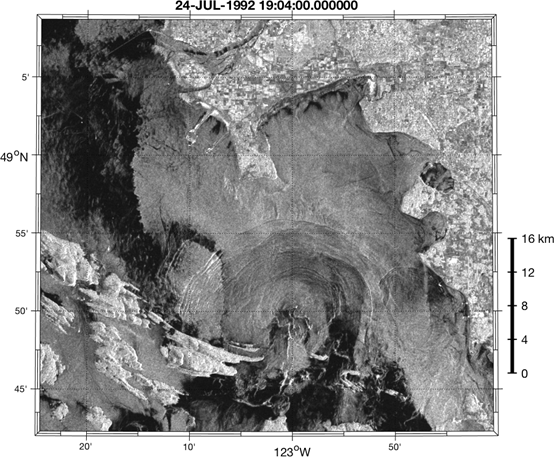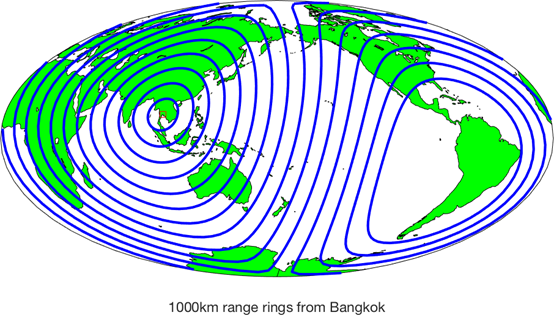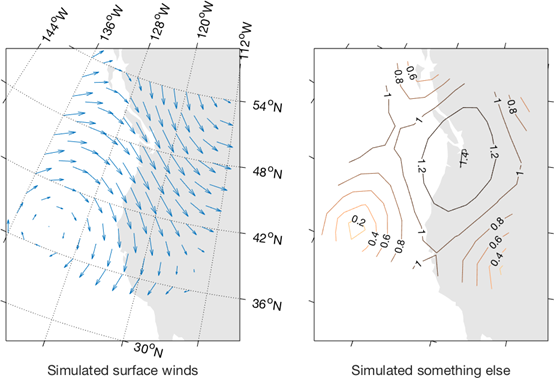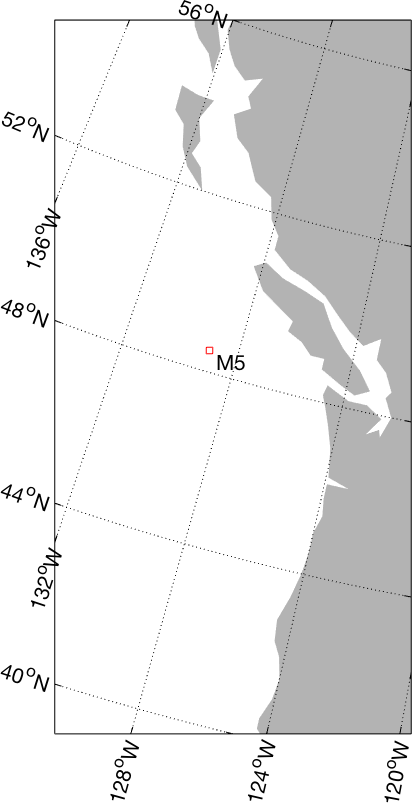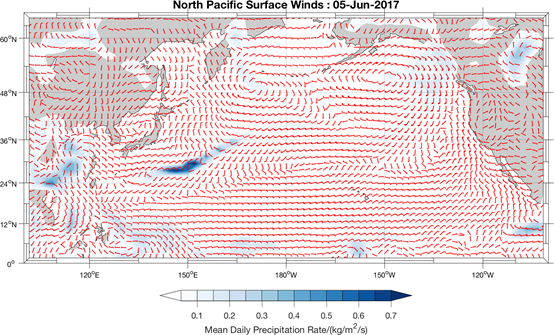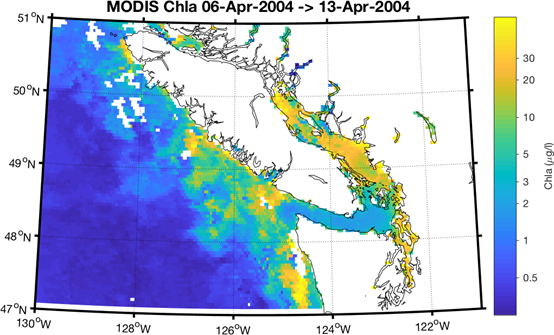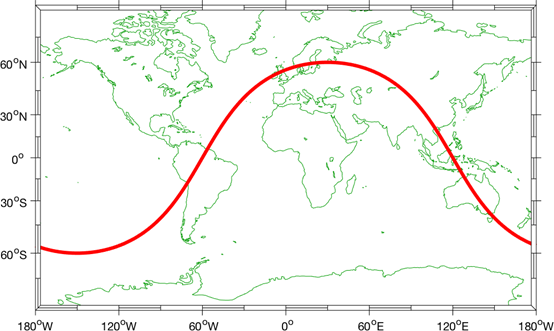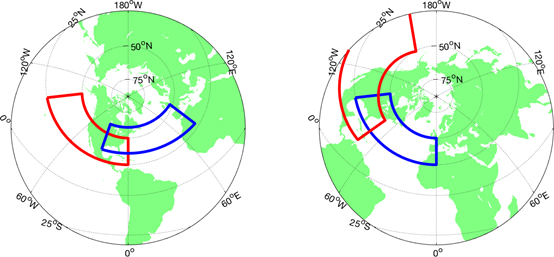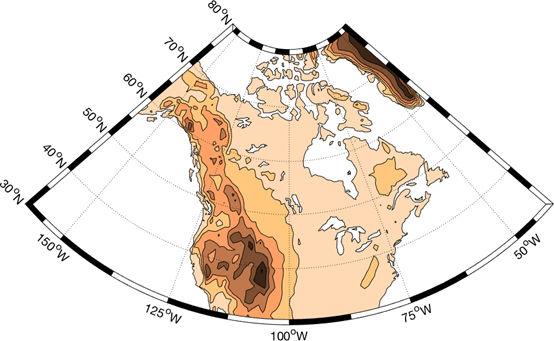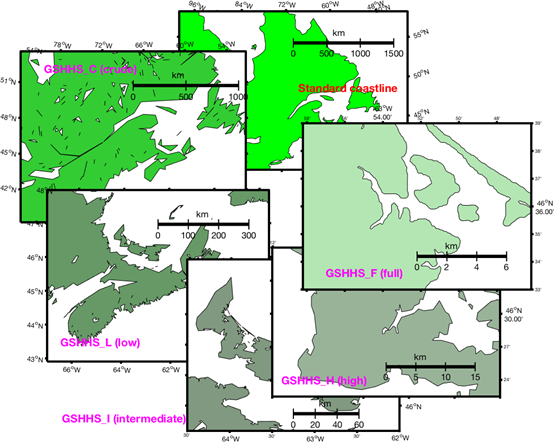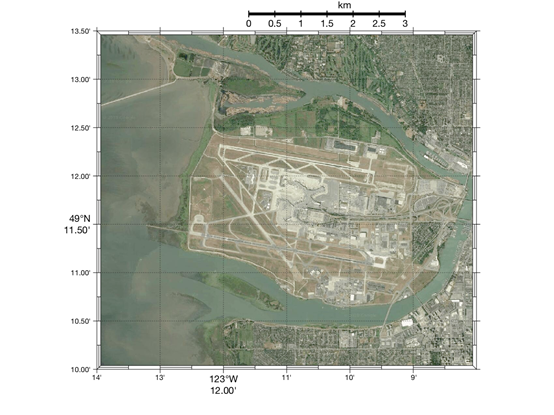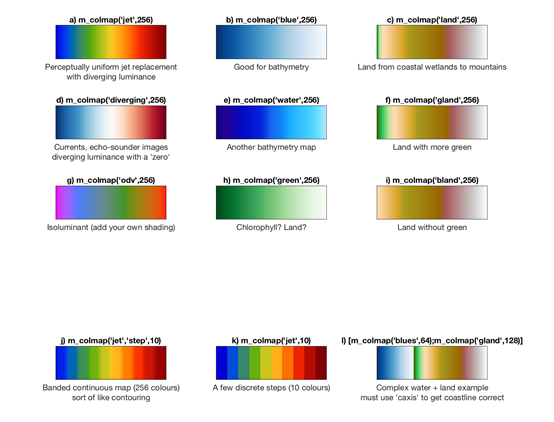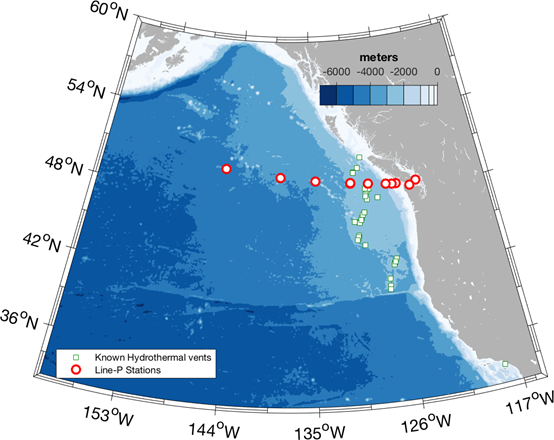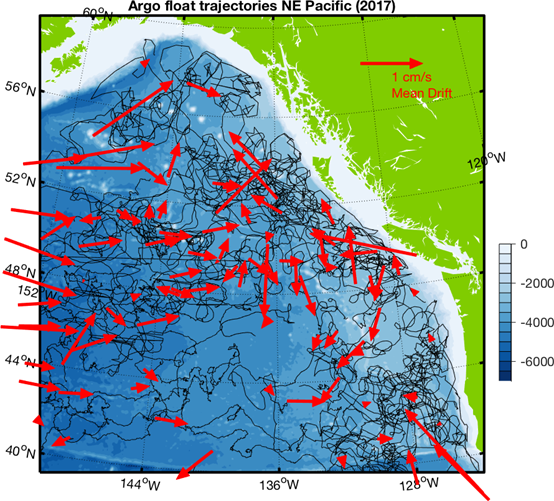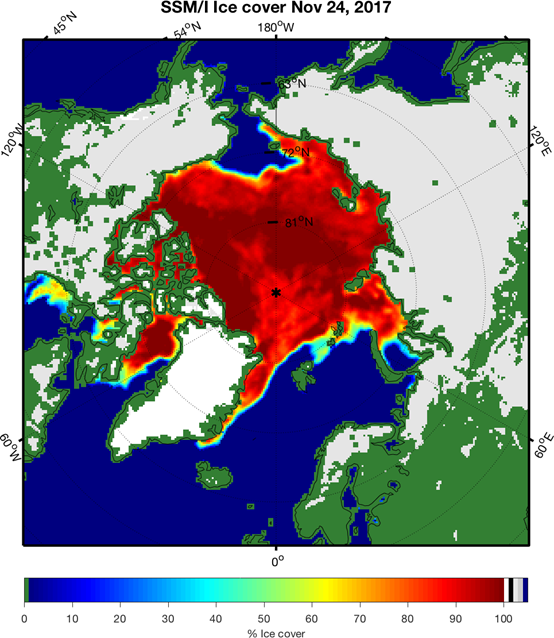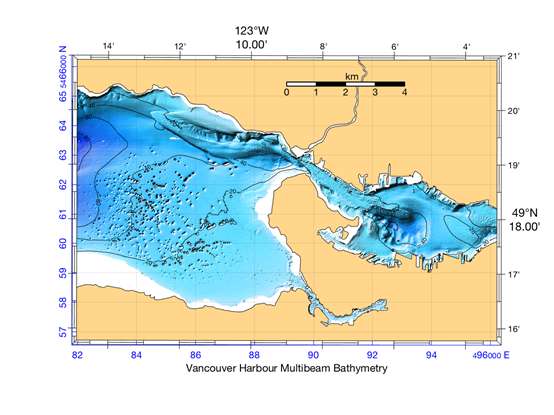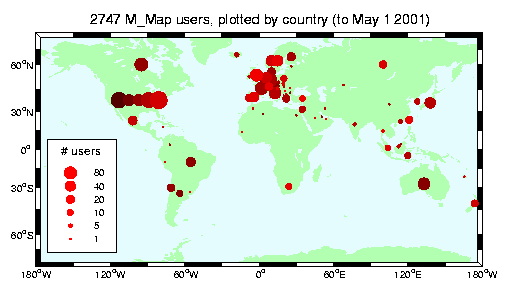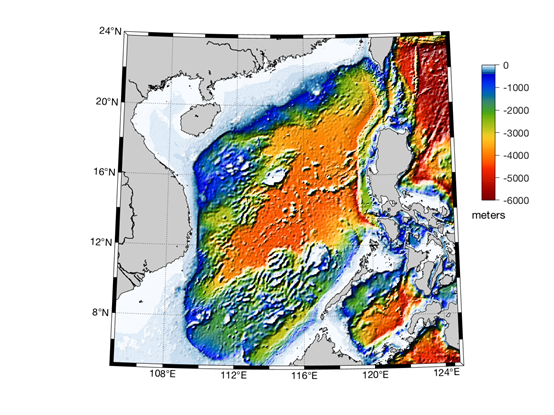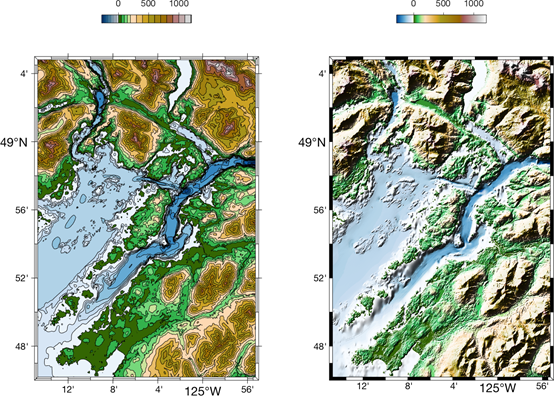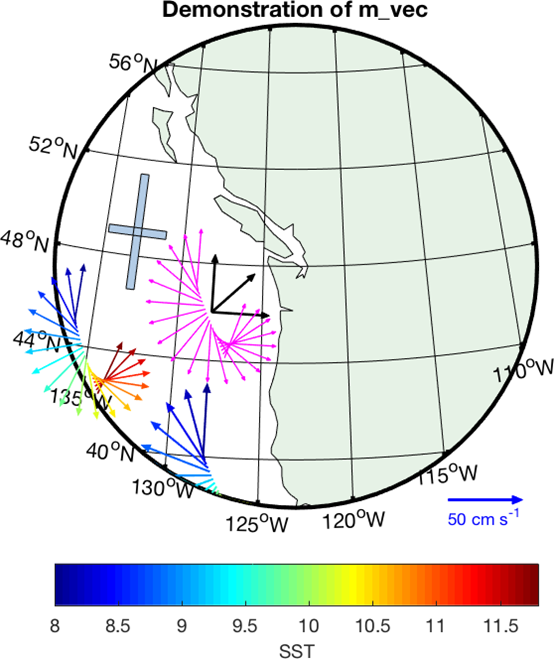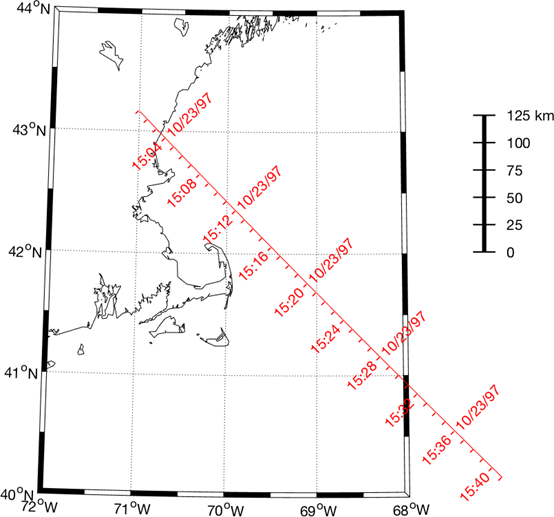欢迎加群获取数据https://www.jianshu.com/p/cb3ccbae9c88

展开全文• ## Matlab画地图之 m_map

千次阅读 2020-08-22 22:07:09
气象家园： https://mp.weixin.qq.com/s/JWi7VllWr93wDw6BStk8Dw M-Map：https://www.eoas.ubc.ca/~rich/map.html ...matlab利用m_map工具包中国地图及散点云图：https://www.cnblogs.com/righdf.
几个缺的图请移驾博客园：cnblogs.com/Gou-Hailong/p/13822460.html

参考：
气象家园： https://mp.weixin.qq.com/s/JWi7VllWr93wDw6BStk8Dw
M-Map：https://www.eoas.ubc.ca/~rich/map.html
中国科学院资源环境数据平台：http://www.resdc.cn/Default.aspx
matlab利用m_map工具包画中国地图及散点云图：https://www.cnblogs.com/righdflf/p/11484189.html
百度经验出图：https://jingyan.baidu.com/article/870c6fc36fdacfb03ee4be58.html

快应用：
shp文件下载：
代码下载：

文章目录1、shp数据1.1 中国科学院资源环境数据1.2 从CSDN上下载的1.3 奇哥给的1.4 数据汇总2、m_map 使用笔记2.1 安装2.2 常用命令3、常用功能实现3.1 画出某省并标出其在中国地图上的位置3.2 用shp文件裁剪图片
1、shp数据
目前搞到的shape文件有：中国科学院资源环境数据、从CSDN上下载的、奇哥给的。
1.1 中国科学院资源环境数据这个数据精度很高，比如，数据2的P2 长度为925，这个数据P2长度是4461；当然精度高同时画起来也超级慢，我的lj 电脑画个图就可以吃一碗泡面了。
它配套一个xls文件：中国各省份与地级市的编码.xls里面存了省市代码。P2数据存在下面的问题：有的省名字缺失，P3数据有的也缺失，但是其对应的代码查询不到，所以不管了。下面补一下P2的省名字。修正后的shp文件名为：sheng.shp。
1.2 从CSDN上下载的L1file='D:\下载\Useful\shp\国家基础地理数据\bou1_4m\bou1_4l.shp';%国界线
P1file='D:\下载\Useful\shp\国家基础地理数据\bou1_4m\bou1_4p.shp';%国界多边形
L2file='D:\下载\Useful\shp\国家基础地理数据\bou2_4m\bou2_4l.shp';%省界线
P2file='D:\下载\Useful\shp\国家基础地理数据\bou2_4m\bou2_4p.shp';%省界多边形
P3file='D:\下载\Useful\shp\国家基础地理数据\bou3_4m\diquJie_polyline.shp';%地市级
L4file='D:\下载\Useful\shp\国家基础地理数据\bou4_4m\BOUNT_line.shp';%县界线
P4file='D:\下载\Useful\shp\国家基础地理数据\bou4_4m\BOUNT_poly.shp';%县界多边形
%读取国界shp文件的内容
bou1_4lx=[L1(:).X];%提取经度信息
bou1_4ly=[L1(:).Y];%提取纬度信息
bou1_4px=[P1(:).X];%提取经度信息
bou1_4py=[P1(:).Y];%提取纬度信息
bou2_4lx=[L2(:).X];%提取经度信息
bou2_4ly=[L2(:).Y];%提取纬度信息
bou2_4px=[P2(:).X];%提取经度信息
bou2_4py=[P2(:).Y];%提取纬度信息
bou3_4px=[P3(:).X];%提取经度信息
bou3_4py=[P3(:).Y];%提取纬度信息
bou4_4lx=[L4(:).X];%提取经度信息
bou4_4ly=[L4(:).Y];%提取纬度信息
bou4_4px=[P4(:).X];%提取经度信息
bou4_4py=[P4(:).Y];%提取纬度信息

figure;
subplot(231);
mapshow(L1,'Color','k');    title('1China mapshow PolyLine-1')
subplot(232);
mapshow(P1,'FaceColor','r');title('1China mapshow Polygon-2')
subplot(233);
geoshow(P2,'FaceColor','y');title('2China geoshow Polygon-3')
subplot(234);
mapshow(L2,'Color','g');    title('2China mapshow PolyLine-4')
subplot(235);
m_proj('miller','lon',[70,140],'lat',[10,60]);%选择投影方式
m_plot(bou3_4px,bou3_4py);%绘国界, 默认黑色,
m_grid;%绘制边框
title('3China m-proj PolyLine-5')
subplot(236);
m_proj('robinson','lon',[70,140],'lat',[10,60]);%选择投影方式
m_plot(bou4_4lx,bou4_4ly,'b');%绘国界，蓝色
m_grid;%绘制边框
title('4China m-proj PolyLine-6')

结果：可以看到地级市只有内部线，想要使用，得和省界线配合使用
L2file='D:\下载\Useful\shp\国家基础地理数据\bou2_4m\bou2_4l.shp';%省界线
L3file='D:\下载\Useful\shp\国家基础地理数据\bou3_4m\diquJie_polyline.shp';%地市级
bou2_4lx=[L2(:).X];%提取经度信息
bou2_4ly=[L2(:).Y];%提取纬度信息
bou3_4lx=[L3(:).X];%提取经度信息
bou3_4ly=[L3(:).Y];%提取纬度信息
m_plot(bou3_4lx,bou3_4ly,'k');
hold on;
m_plot(bou2_4lx,bou2_4ly,'linewidth',3,'color','k');1.3 奇哥给的
奇哥给的和上面那个是一样的，多了世界国家。下面将两个整合到一起。代码用的是 3 中的功能1，然后将 NAME 改成 FCNAME 即可。调用：
Sjfile='D:\下载\Useful\shp\国家基础地理数据\世界国家\世界国家.shp';
drawsheng(Sjfile,'中国');

1.4 数据汇总

文件名
描述

bou1_4m
中国国界

bou2_4m
中国省界

bou3_4m
中国地市级（只有内部线）

bou4_4m
中国县级

hyd1_4m
中国一级河流

hyd2_4m
中国二级河流

hyd4_4m
中国四级河流

hyd5_4m
中国五级河流

rai_4m
中国主要铁路

res1_4m
省会标示

res2_4m
地市级以上居民地

res4_4m
县以上居民地级

roa_4m
中国主要公路

世界国家
世界国家

省界
另一个省界（不用）

中国省级行政区划_shp.rar
一般不用

县级_截止08年_shp.rar
一般不用

sheng
中国省界（精度高，慢）含修正后的

shi
中国市界（精度高，慢）

常用数据路径汇总：
L1file='D:\下载\Useful\shp\国家基础地理数据\bou1_4m\bou1_4l.shp';%国界线
P1file='D:\下载\Useful\shp\国家基础地理数据\bou1_4m\bou1_4p.shp';%国界多边形
L2file='D:\下载\Useful\shp\国家基础地理数据\bou2_4m\bou2_4l.shp';%省界线
P2file='D:\下载\Useful\shp\国家基础地理数据\bou2_4m\bou2_4p.shp';%省界多边形
L3file='D:\下载\Useful\shp\国家基础地理数据\bou3_4m\diquJie_polyline.shp';%地市级
L4file='D:\下载\Useful\shp\国家基础地理数据\bou4_4m\BOUNT_line.shp';%县界线
P4file='D:\下载\Useful\shp\国家基础地理数据\bou4_4m\BOUNT_poly.shp';%县界多边形
P03file='D:\下载\Useful\shp\中国科学院资源环境数据\shi\CN-shi-A.shp';%地级市
P021file='D:\下载\Useful\shp\中国科学院资源环境数据\sheng\CN-sheng-A.shp';%省
P02file='D:\下载\Useful\shp\中国科学院资源环境数据\sheng\sheng.shp';%修正之后的省
Sjfile='D:\下载\Useful\shp\国家基础地理数据\世界国家\世界国家.shp';

%读取国界shp文件的内容
bou1_4lx=[L1(:).X];%提取经度信息
bou1_4ly=[L1(:).Y];%提取纬度信息
bou1_4px=[P1(:).X];%提取经度信息
bou1_4py=[P1(:).Y];%提取纬度信息
bou2_4lx=[L2(:).X];%提取经度信息
bou2_4ly=[L2(:).Y];%提取纬度信息
bou2_4px=[P2(:).X];%提取经度信息
bou2_4py=[P2(:).Y];%提取纬度信息
bou3_4lx=[L3(:).X];%提取经度信息
bou3_4ly=[L3(:).Y];%提取纬度信息
bou4_4lx=[L4(:).X];%提取经度信息
bou4_4ly=[L4(:).Y];%提取纬度信息
bou4_4px=[P4(:).X];%提取经度信息
bou4_4py=[P4(:).Y];%提取纬度信息

下面对中国行政单位划分做个总结：

https://www.cnblogs.com/Gou-Hailong/p/13545885.html
详细描述请移驾：https://jingyan.baidu.com/article/59a015e398ed3ff794886589.html
行政区划代码查询：https://xingzhengquhua.51240.com/

2、m_map 使用笔记
2.1 安装

下载链接：https://www.eoas.ubc.ca/~rich/map.html下好工具箱解压后，将文件夹放在一个不常动的地方，我放在了D:\MATLAB\R2017a\toolbox中，然后打开matlab，点击set path(设置路劲)，将m_map路径加进去就行了。测试是否成功的话，就在matlab命令空间敲m_demo(1)括号里面可以是1-15，这实际上m_map中附带的一个函数，在文件m_demo.m，看看可不可以画出来图。
2.2 常用命令

User Guide：https://www.eoas.ubc.ca/~rich/mapug.html#p2

m_proj get //得到当前地图的投影方式
m_proj('set','xxx'); //xxx见下表，查看投影方式的细节
m_proj('xxx');       //设置当前投影方式
m_scale(250000);     //比例尺设成1:250000
m_scale('auto');     //默认比例尺
a=m_scale            //返回当前比例尺
m_coord('IGRF-geomagnetic',datenum(2000,1,1)); //设置IGRF-2000
m_coast('line', ...optional line arguments );
/*m_coast函数可以访问M_Map数据库。
将海岸线绘制为简单的线条。
可选参数是线的样式、宽度、颜色等。
*/
m_coast('patch', ...optional patch arguments );
m_coast('patch',[.7 .7 .7],'edgecolor','g');//绘制灰色土地，绿色勾画
m_coast('speckle', ....optional m_hatch arguments);

m_elev('contour',LEVELS,'edgecolor','b');//画轮廓
m_elev('contourf',LEVELS, optional contourf arguments);//填充轮廓
[Z,LONG,LAT]=m_elev([LONG_MIN LONG_MAX LAT_MIN LAT_MAX]);//返回深度Z的矩形矩阵的位置,经纬度

m_plot(LONG,LAT,...line properties)      % draw a line on a map (erase current plot)
m_plot(bou2_4lx,bou2_4ly,'linewidth',3,'color','k');
m_line(LONG,LAT,...line properties)      % draw a line on a map
m_text(LONG,LAT,'string')                % Text
m_quiver(LONG,LAT,U,V,S)                 % A quiver plot
m_patch(LONG,LAT,..patch properties)     % Patches.
m_annotation('line',LON,LAT)             % Annotation

可用的投影方式有：

参数
中文描述
图

Stereographic
保角，常用于极区

Orthographic
既不是等面积也不是保角的，而是类似于地球的透视图Azimuthal Equal-area
朗伯方位等面积投影Azimuthal Equidistant
等距

Gnomonic
点切投影，地图上所有的直线(不仅仅是那些通过中心的)都是大圆路线

Satellite
地球的透视图，由卫星在特定的高度所看到。指定视点高度而不是为地图指定半径

Albers Equal-Area Conic
等积阿波斯圆锥

Lambert Conformal Conic
兰伯特等角圆锥Mercator
墨卡托，正形切圆柱

Miller Cylindrical
米勒圆柱Equidistant Cylindrical
等距圆柱

Cylindrical Equal-Area
等积圆柱

Oblique Mercator
斜轴墨卡托Transverse Mercator
横轴墨卡托

Sinusoidal
伪圆柱投影

Gall-Peters
纬线和子午线的平行线都呈现为直线，但垂直尺度被扭曲

Hammer-Aitoff
具有弯曲子午线和平行线的等面积投影Mollweide
外德投影，也称为椭圆或等积投影。在这个投影中，平行线是直的。Robinson
洛滨孙，养眼UTM
UTM投影注：本人自己翻译，有的没见过，可能不准，图片来源于上述链接。

通常，全世界的地图都是墨卡托（Mercator），尽管米勒圆柱投影通常看起来更好，因为它没有强调极地区域。 另一个选择是Hammer-Aitoff或Mollweide（使子午线在两极附近弯曲在一起）。 两者都是等面积的。 将这些投影用于在中点附近没有赤道的地图可能不是一个好主意。 Robinson投影不是等面积或等角投影，而是国家地理杂志的选择（无论如何），并且也出现在IPCC报告中。
如果您要绘制的东西的北/南范围较大，但不是很宽（例如北美洲和南美洲，或者北大西洋和南大西洋），那么正弦或Mollweide投影将看起来非常不错。 另一个选择是“横轴墨卡托”，尽管通常只用于非常大比例尺的地图（即，覆盖很小面积的地图）。
对于一个半球或其他半球内较小的区域（例如，澳大利亚，美国，地中海，北大西洋），您可能会选择圆锥投影。 两种可用的圆锥投影之间的差异非常细微，如果您对投影不太了解，那么使用哪种圆锥投影可能不会有太大的区别。
如果您要画的区域变得更小，则使用哪种投影都无关紧要。 我认为在很多情况下有用的一种投影是斜墨卡托，因为您可以将其沿着长（但窄）的沿海地区对齐。 如果沿着经度/纬度线的地图限制正常，请使用横轴墨卡托或圆锥投影。 如果要以米为单位建立网格，则UTM投影也很有用，因为投影坐标（即“东和北”）以米为单位。
极地地区传统上是使用Stereographic投影来绘制地图的，因为每个人似乎都认为具有“靶心”式的纬线模式看起来不错（经度线在极点汇聚在一起的事实也使它们成为极地科学家几乎不可抗拒的目的地 和探险者）。

3、常用功能实现
3.1 画出某省并标出其在中国地图上的位置

全代码：https://blog.csdn.net/Gou_Hailong/article/details/108209764

调用实例：
file='D:\下载\Useful\shp\国家基础地理数据\bou2_4m\bou2_4p.shp';%省界多边形
str='广东省';
drawsheng(file,str);
shapewrite(sheng,filename); //存到shp文件中
> 函数全代码请移驾：[https://blog.csdn.net/Gou_Hailong/article/details/108209395](https://blog.csdn.net/Gou_Hailong/article/details/108209395)

3.2 用shp文件裁剪图片
先搞个简单的：河南省的
1.第一步，把河南省的shp提取出来，注意看其范围
P2file='D:\下载\Useful\shp\国家基础地理数据\bou2_4m\bou2_4p.shp';%省界多边形
henan=drawsheng(P2file,'河南省');%第一步，把河南省的shp提取出来，注意看其范围可以看到，其大致范围是110°E-117°E, 30°N-37°N。

2.从 tif 里面将该大致范围搞出来Henan，并提取shp边界xv, yv，之后用inpolygon搞出逻辑矩阵in(在边界内的为1)
TDMSPfile='D:\复习资料\自学\7_科研\夜光遥感\data\DMSP\F121999.v4\F121999.v4b_web.stable_lights.avg_vis.tif';
Henan=TDMSP(38*120:45*120,290*120:297*120);%根据自己需要裁减
X=30:0.0083333333:37;
Y=110:0.0083333333:117;
[a,b]=size(Henan);
X1=repmat(X',1,b);
Y1=repmat(Y,a,1);
X1=flipud(X1);%这个得上下翻转一下。
xv=henan.X;yv=henan.Y;        %提取边界
xv = xv(1:end-1); yv = yv(1:end-1);
in = inpolygon(Y1,X1,xv,yv);    %返回逻辑矩阵，


3.把边界外的数据搞成nan
photo=zeros(a,b);
photo(:)=nan;
for i=1:a
for j=1:b
if in(i,j)
photo(i,j)=Henan(i,j);
end
end
end  %在边界外的搞成nan


4.画图
[x,x1,y,y1] = getxy(Y,X);
x=(x-110).*120;
y=(y-30).*120;
photo=flipud(photo);%contourf 上下翻转一下，才变成imshow
contourf(photo,'LineStyle','none');
colormap(jet);colorbar
set(gca,'XTick',x,'XTicklabel',x1);   %设置x,y轴
set(gca,'YTick',y,'YTicklabel',y1);
title('河南省夜光遥感影像');

colormap(gray);colorbar  //宁夏的,PS：出逻辑矩阵的时候超级慢，这还是一个简单的边界，目前只能这么搞了，还没有其他方法。
跑全中国的跑十几分钟还跑步不来。我丢，中国大陆矩阵大小4081x7561 = 30,856,441，本来以为是三百多万，从8.23 14:50开始跑，跑到8.23 23:02跑了177万，我觉得明天早上应该就跑完了，有点期待夹杂着莫名的兴奋。到了8.24 7:57跑到了270万，我想着在有俩小时估计就跑完了，但是10点之后，我发现还没跑完，跑到了310万。我去，不是308万吗，又仔细数了数零，发现是3080万，当场哭晕，那不得10天吗，就没管它，它自己在那跑，到8.24 16:03跑到390万停，结果如下图：算是把雄鸡鸡冠画出来了吧。。

突然想到一个新方法，中国边界内的最大内接圆一定在中国里面，中国边界的最小外接圆一定在中国外边，所以最后只需判断中间夹的那个小圆环就好了。这是裁北京市的图，小圆太小，大圆太大，效果还是不太理想，求重心求得精确一点就好了。改进了求重心的算法，效果如上图（右）还可以。
这个功能的算法，目前来说，我是没招了，全代码链接如下：

https://blog.csdn.net/Gou_Hailong/article/details/108209764

但是，对于中国大陆来说，两个圆还是有点少：上图虽简单，但上面花了我好几个小时，搞出来了这7个圆的信息

R
X
Y
信息

11.807875718188898
1.338997219786234e+02
30.905277734156662
内部不要

31.334089276544790
1.044958332073500e+02
43.506944350416660
外部不要

13.553968551057642
1.338997219786233e+02
22.504166656650000
内部不要

11.586902594103240
1.044958332073500e+02
53.999999864000000
内部不要

9.333210635315217
1.086963887461033e+02
33.005555503533330
内部要

4.040350856671964
1.254986109011167e+02
45.607222119793335
内部要

8.597190190877908
87.693611052336680
37.206111042286665
内部要我梦寐以求的小公鸡，快点出来吧，这是用mapshow(shp)画出来的。2020/8/24 20:34 还在苦逼等待程序运行中…（真累，明天换个活，先歇歇。）
2020/8/26 16:13 经历了大约两天时间，终于跑完了，加上那七个圆，算法运行时间大大减少了，之前的话少说也得10天，现在大约两天跑好了，电脑烫的厉害。下面看下结果：
Elapsed time is 169864.909166 seconds.
169864.909166s = 47.18 h
------------1------------------
8.23 14.50 开始跑
8.23 20.05 1334000
8.23 23:02 1777000
8.24 7.57 2722000
8.24 14.30 374万
8.24 16.03 390万停
------------2------------------
8.24 14.22 开始跑
8.24 14.25 700多万，慢起来了
8.24 14.30 766万
8.24 16.02 1060万
蹦了，程序错了
------------3------------------
8.24 17:00 start
8.24 22:18 307w
8.25 6:45 713w
8.25 12:00 1000w
8.25 16:23 1239w, 做个备份，photo1  i=1638, j=6164
8.25 22:36 1905w, 做个备份，photo2  i=2520, j=664
8.26 8:53 2737w, 做个备份，photo3  i=3620, j=3749
8.26 16:03 ok;
--------历时-47.18 h--------这张图片具有重大的“历史纪念”意义，希望CSDN不要和谐掉。。


展开全文• 1、全代码function varargout = getxy(varargin)%% 此函数用来搞定画地图时，经纬度坐标注释% 输入：% XArray：经度数组% YArray：纬度数组% d： 显示几个数% 输出：% x： 经度数组，数字% x1： 经度数组，字符% y：...
1、全代码function varargout = getxy(varargin)%% 此函数用来搞定画地图时，经纬度坐标注释% 输入：%   XArray：经度数组%   YArray：纬度数组%   d：     显示几个数% 输出：%   x：     经度数组，数字%   x1：    经度数组，字符%   y：     纬度数组，数字%   y1：    纬度数组，字符%   ex：    经纬度极值：经大小，纬大小% 使用示例：% [x,x1,y,y1]=getxy(XArray,YArray,d);%   [x,x1,y,y1]=getxy(XArray,YArray);%   [x,x1,y,y1,ex]=getxy(XArray,YArray);%   XArray,YArray 东正西负，北正南负%-------------------------------------------------------------------%%%%    Authors:   Bill O'Hanlon%%%%    EMAIL:     ohanlon@qq.com%%%%    DATE:      24-08-2020%% 输入判断if nargin==2XArray=varargin{1};YArray=varargin{2};d=5;elseif nargin==3XArray=varargin{1};YArray=varargin{2};d=varargin{3};elsedisp('输入参数过多或过少');return;end%% 求极值，并划分Xmax=max(XArray);Xmin=min(XArray);Ymax=max(YArray);Ymin=min(YArray);extreme=zeros(4,1); %极值数组，存放极大极小值Xmax=ceil(Xmax);Ymax=ceil(Ymax);Xmin=floor(Xmin);Ymin=floor(Ymin);extreme(1)=Xmax;extreme(2)=Ymax;extreme(3)=Xmin;extreme(4)=Ymin;detX=Xmax-Xmin;detY=Ymax-Ymin;dX=ceil(detX/d);dY=ceil(detY/d);x=Xmin+dX:dX:Xmax;y=Ymin+dY:dY:Ymax;%% 将划分好的转化为字符，并加°和S/N/W/Ex1=sprintfc('%g',x);%转成字符串数组y1=sprintfc('%g',y);%转成字符串数组n1=size(x1,2);n2=size(y1,2);for i=1:n1if x(i)>=0x1{1,i}=[x1{1,i},'°E'];elsex1{1,i}=x1{1,i}(2:end);x1{1,i}=[x1{1,i},'°W'];endendfor i=1:n2if y(i)>=0y1{1,i}=[y1{1,i},'°N'];elsey1{1,i}=y1{1,i}(2:end);y1{1,i}=[y1{1,i},'°S'];endendvarargout{1}=x;varargout{2}=x1;varargout{3}=y;varargout{4}=y1;if nargout==5varargout{5}=extreme;endend2、调用subplot(121);% 下面搞定横纵坐标注释XArray=[P2(:).X];%所有多边形的经度YArray=[P2(:).Y];%%所有多边形的纬度[x,x1,y,y1]=getxy(XArray,YArray);disp('drawing...');mapshow(P2,'FaceColor','w');mapshow(sheng,'FaceColor','r');set(gca,'XTick',x,'XTicklabel',x1);   %设置x,y轴set(gca,'YTick',y,'YTicklabel',y1);box on;title([str '的位置']);axis equalsubplot(122);% 下面搞定横纵坐标注释XArray=[sheng(:).X];%所有多边形的经度YArray=[sheng(:).Y];%%所有多边形的纬度[x,x1,y,y1,ex]=getxy(XArray,YArray);mapshow(sheng,'FaceColor','r');set(gca,'XTick',x,'XTicklabel',x1);   %设置x,y轴set(gca,'YTick',y,'YTicklabel',y1);box on;title([str '行政规划图']);axis equal结果：展开全文• %% 此函数用来搞定画地图时，经纬度坐标注释 % 输入： % XArray：经度数组 % YArray：纬度数组 % d： 显示几个数 % 输出： % x： 经度数组，数字 % x1： 经度数组，字符 % y： 纬度数组，数字 % y1： 纬度数组，字符...
1、全代码
function varargout = getxy(varargin)
%% 此函数用来搞定画地图时，经纬度坐标注释
% 输入：
%   XArray：经度数组
%   YArray：纬度数组
%   d：     显示几个数
% 输出：
%   x：     经度数组，数字
%   x1：    经度数组，字符
%   y：     纬度数组，数字
%   y1：    纬度数组，字符
%   ex：    经纬度极值：经大小，纬大小
% 使用示例：
% 	[x,x1,y,y1]=getxy(XArray,YArray,d);
%   [x,x1,y,y1]=getxy(XArray,YArray);
%   [x,x1,y,y1,ex]=getxy(XArray,YArray);
%   XArray,YArray 东正西负，北正南负
%-------------------------------------------------------------------
%%%%    Authors:   Bill O'Hanlon
%%%%    EMAIL:     ohanlon@qq.com
%%%%    DATE:      24-08-2020
%% 输入判断
if nargin==2
XArray=varargin{1};
YArray=varargin{2};
d=5;
elseif nargin==3
XArray=varargin{1};
YArray=varargin{2};
d=varargin{3};
else
disp('输入参数过多或过少');
return;
end
%% 求极值，并划分
Xmax=max(XArray);
Xmin=min(XArray);
Ymax=max(YArray);
Ymin=min(YArray);
extreme=zeros(4,1); %极值数组，存放极大极小值
Xmax=ceil(Xmax);
Ymax=ceil(Ymax);
Xmin=floor(Xmin);
Ymin=floor(Ymin);

extreme(1)=Xmax;
extreme(2)=Ymax;
extreme(3)=Xmin;
extreme(4)=Ymin;
detX=Xmax-Xmin;
detY=Ymax-Ymin;
dX=ceil(detX/d);
dY=ceil(detY/d);
x=Xmin+dX:dX:Xmax;
y=Ymin+dY:dY:Ymax;
%% 将划分好的转化为字符，并加°和S/N/W/E
x1=sprintfc('%g',x);%转成字符串数组
y1=sprintfc('%g',y);%转成字符串数组
n1=size(x1,2);n2=size(y1,2);
for i=1:n1
if x(i)>=0
x1{1,i}=[x1{1,i},'°E'];
else
x1{1,i}=x1{1,i}(2:end);
x1{1,i}=[x1{1,i},'°W'];
end
end
for i=1:n2
if y(i)>=0
y1{1,i}=[y1{1,i},'°N'];
else
y1{1,i}=y1{1,i}(2:end);
y1{1,i}=[y1{1,i},'°S'];
end
end
varargout{1}=x;
varargout{2}=x1;
varargout{3}=y;
varargout{4}=y1;
if nargout==5
varargout{5}=extreme;
end
end

2、调用
subplot(121);
% 下面搞定横纵坐标注释
XArray=[P2(:).X];%所有多边形的经度
YArray=[P2(:).Y];%%所有多边形的纬度
[x,x1,y,y1]=getxy(XArray,YArray);
disp('drawing...');
mapshow(P2,'FaceColor','w');
mapshow(sheng,'FaceColor','r');
set(gca,'XTick',x,'XTicklabel',x1);   %设置x,y轴
set(gca,'YTick',y,'YTicklabel',y1);
box on;
title([str '的位置']);
axis equal

subplot(122);
% 下面搞定横纵坐标注释
XArray=[sheng(:).X];%所有多边形的经度
YArray=[sheng(:).Y];%%所有多边形的纬度
[x,x1,y,y1,ex]=getxy(XArray,YArray);
mapshow(sheng,'FaceColor','r');
set(gca,'XTick',x,'XTicklabel',x1);   %设置x,y轴
set(gca,'YTick',y,'YTicklabel',y1);
box on;
title([str '行政规划图']);
axis equal

结果：CSDN 脑子秀逗了，说图片违规，dd，
博客园链接：https://www.cnblogs.com/Gou-Hailong/p/13559123.html

V2.0
2020.11.1，今天调用这个函数的时候，发现一个问题。就是，x,y这俩是数据，x1，y1这俩是与其对应的字符串；但是，set(gca,'XTick',x,'XTicklabel',x1);这个代码里面的x是矩阵对应的列数，这个列数并不是x保存的数据，所以更新了一下这个函数。我将此版本称为V1.0，现贴在下面（第一个版本对应一系列函数，不能改，否则那些函数就塌了）：
function varargout = getxy(varargin)
%% 此函数用来搞定画地图时，经纬度坐标注释
% 输入：
%   XArray：经度数组
%   YArray：纬度数组
%   d：     显示几个数
% 输出：
%   x：     经度数组，数字
%   x1：    经度数组，字符
%   y：     纬度数组，数字
%   y1：    纬度数组，字符
%   ex：    经纬度极值：经大小，纬大小
% 使用示例：
% 	[x,x1,y,y1]=getxy(XArray,YArray,d);
%   [x,x1,y,y1]=getxy(XArray,YArray);
%   [x,x1,y,y1,ex]=getxy(XArray,YArray);
%   XArray,YArray 东正西负，北正南负
%-------------------------------------------------------------------
%%%%    Authors:   Bill O'Hanlon
%%%%    EMAIL:     ohanlon@qq.com
%%%%    DATE:      01-11-2020
%% 输入判断
if nargin==2
XArray=varargin{1};
YArray=varargin{2};
d=5;
elseif nargin==3
XArray=varargin{1};
YArray=varargin{2};
d=varargin{3};
else
disp('输入参数过多或过少');
return;
end
%% 求极值，并划分
Xmax=max(XArray);
Xmin=min(XArray);
Ymax=max(YArray);
Ymin=min(YArray);
extreme=zeros(4,1); %极值数组，存放极大极小值
Xmax=ceil(Xmax);
Ymax=ceil(Ymax);
Xmin=floor(Xmin);
Ymin=floor(Ymin);

extreme(1)=Xmax;
extreme(2)=Ymax;
extreme(3)=Xmin;
extreme(4)=Ymin;
detX=Xmax-Xmin;
detY=Ymax-Ymin;
dX=ceil(detX/d);
dY=ceil(detY/d);
x=Xmin+dX:dX:Xmax;
y=Ymin+dY:dY:Ymax;
%% 将划分好的转化为字符，并加°和S/N/W/E
x1=sprintfc('%g',x);%转成字符串数组
y1=sprintfc('%g',y);%转成字符串数组
n1=size(x1,2);n2=size(y1,2);
for i=1:n1
if x(i)>=0
x1{1,i}=[x1{1,i},'°E'];
else
x1{1,i}=x1{1,i}(2:end);
x1{1,i}=[x1{1,i},'°W'];
end
end
for i=1:n2
if y(i)>=0
y1{1,i}=[y1{1,i},'°N'];
else
y1{1,i}=y1{1,i}(2:end);
y1{1,i}=[y1{1,i},'°S'];
end
end
xstep=XArray(2)-XArray(1);  %得到俩数组的步长
ystep=YArray(2)-YArray(1);
x=(x-Xmin)./xstep;          %得到x1,y1对应的整数。
y=abs((y-Ymin))./ystep;
varargout{1}=x;
varargout{2}=x1;
varargout{3}=y;
varargout{4}=y1;
if nargout==5
varargout{5}=extreme;
end

end



展开全文• %生成背景地图地图 h = worldmap(‘France’); %读取和显示大陆架 landareas = shaperead(‘landareas.shp’,‘UseGeoCoords’, true); geoshow (landareas, ‘FaceColor’, [1 1 .5]); %埃菲尔铁塔的坐标 TowerLon ...
• MATLAB绘制地图课件1使用向量绘制地图1.1绘制全球海岸线向量数据可以表示一个地图。这种向量存在的形式是一系列的经纬度或投影坐标对，它们代表一个点集、一个线条或者多边形。例如，描绘出行政区域边界的点、公路...
• matlab画地图的工具：worldmap和m_map*用MATLAB画世界地图并加散点clear;clc;worldmap([-55 -30],[-110 -60]);h1=axesm('MapProjection','eqdcylin','maplatlimit',[-58-30],'maplonlimit',[-110-60],'frame','on','...
• 1、用matlab画世界地图[matlab自带的例子]ax = worldmap('World'); setm(ax, 'Origin', [0 180 0]) land = shaperead('landareas', 'UseGeoCoords', true); geoshow(ax, land, 'FaceColor', [0.5 0.7 0.5]) lakes = ...
• 目前暂时没有大佬用matlab绘制疫情地图，其实MATLAB绘制地图还是相对容易的，于是就拿了两个小时，专门研究了一下，遂成此文。 一、准备工作 开始之前需要准备好malab，中国地图shp文件，m_map工具包。 中国地图...
• 1使用向量绘制地图1.1绘制全球海岸线向量数据可以表示一个地图。这种向量存在的形式是一系列的经纬度或投影坐标对，它们代表一个点集、一个线条或者多边形。例如，描绘出行政区域边界的点、公路系统、城市的中心或者...
• 开始之前需要准备好...中国地图shp文件可以在下面的链接中下载：本文借鉴了下面链接中教程，该方法为matlab自带的画图工具包绘制方法，在我电脑上geoshow命令运行时间特别长，不知道为什么，感兴趣的同学可以试试：...
• 利用matlab画中国地图的几种方法1、下载 arc_map.zip, 内有/shape_files/china.shp 文件，地址如下：http://venus.unive.it/matdid.php?utente=smagrini&base=ec++urbana+e+regionale+-+aa+2008-2009%2Fprimo+......

# matlab画地图matlab 订阅